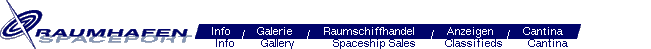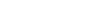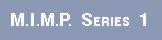Dear MIMP collectors: This page was first created already in the late 90s. Not much was know back then about all the MIMP variations. Since that time many color variants have been found. This page will not be maintained as a source for all known variations. I recently added subjective information about rare neon variants as a final update to this page in January 2016. Happy collecting, Wolfgang

Series 1 monsters (#1-48) first came in four colors: light olive, red, yellow, dark purple. #1-24 were available in two different colors of these, #25-48 in all four different. Later four "new hot colors" were added: neon green, light red, neon yellow, light purple. First it seemed that #1-24 exist in their corresponding new brighter colors and #25-48 exist in all four "new hot colors". Now it's clear that all Series 1 figures exist in all four neon colors. Rare neon color variants were released country specific e.g. in France or Italy. Rare neon colors typically are those where the corresponding classic colors don't exist.

Some figures were also available in other colors, these came with the Board Game, Nabisco Frosted Wheat Squares or the Monster Rock Music Cassette. Those colors are cyan, orange, dark green, and magenta.

Some more color information from ://monsterinmypocket.wikia.com/wiki/Series_1 :
Releases in other countries included a number of unusual colours. Italian releases came in blue, orange-red, yellow, neon green and gold, while Mexican, Argentine and Peruvian figures included a large array of colours including black, white, clear or translucent. Argentine and Peruvian figures tended to be of a lower quality than the European and North American releases.

The Series 1 monsters were available in 1-packs, 4-packs, 12-packs and 24-pack display cases. The two different 24-packs had all 48 monsters, one the odd-numbered monsters, the other the even-numbered monsters.

Series 1, glowing in the dark figures

Here are pictures of some packs:

Series 1 4-pack (US)

Series 1 4-pack (Europe)

Series 1 12-pack (US)

Series 1 12-pack (Europe)

Series 1 12-pack "new hot colors" (US)

Series 1 24-pack A (US)

Series 1 24-pack B (US)

Series 1 24-pack A (US) "New hot Colors"

Series 1 Single Pack from Italy

 lightolive red yellow darkpurple neongreen light red neonyellow"chartreuse" lightpurple"thistle" 1. Great Beast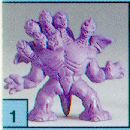x - - x x R R x 2. Hydra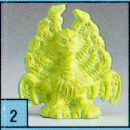x - - x x R R x 3. Werewolf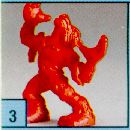x x - - x x R R 4. Behemoth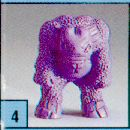- x - x R x R x 5. Griffinx - - x x R x x 6. Tyrannosaurus Rex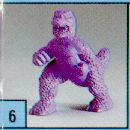x - - x x R R x 7. Cockatricex x - - x x x x 8. Cyclops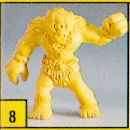- - x x R R x x 9. Tengu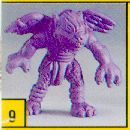- x - x R x R x 10. Triton *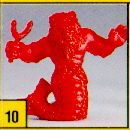- x x - x x x x 11. Kraken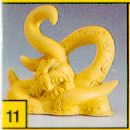- x x - R x x R 12. Jotun Troll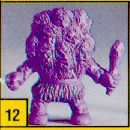- - x x R R x x 13. The Monster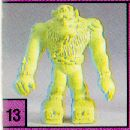x x - - x x R R 14. Manticore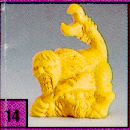- x x - R x x R 15. Karnak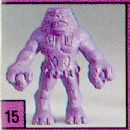- - x x x R x x 16. Coatlicue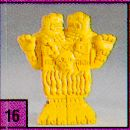- x x - R x x R 17. Bigfootx - - x x R R x 18. Baba Yaga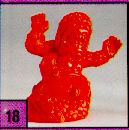x x - - x x R R 19. Kalix - mc x x x x x 20. Catoblepas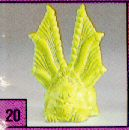x mc x x x x x x 21. Harpyx - - x x x x x 22. Haniver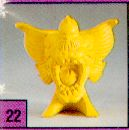- - x x x x x x 23. Hobgoblinx x - - x x R R 24. Windigox x - - x x x x 25. Red Cap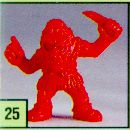x x x x R x x x 26. Medusax x x x x x x x 27. Goblinx x x x x R x x 28. Cerberus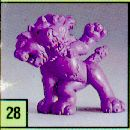x x x x x x x x 29. Zombie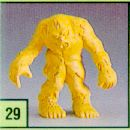x x x x x x x x 30. Chimerax x x x x x x x 31. Ghostx x x x x x x x 32. Ogre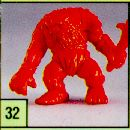x x x x x x R x 33. Vampire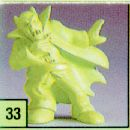x x x x x x R x 34. Roc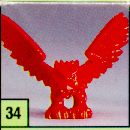x x x x x x R x 35. Gremlinx x x x x R x x 36. Vampiressx x x x x x x x 37. Ghoul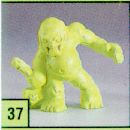x x x x x x x x 38. The Phantom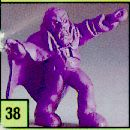x x x x x x x x 39. Mad Scientist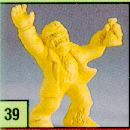x x x x x x x x 40. Winged Panther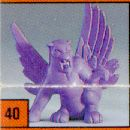x x x x x x x x 41. Mummyx x x x x x x x 42. Charonx x x x x x x x 43. The Beast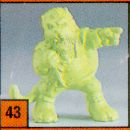x x x x x x x x 44. Witch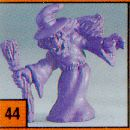x x x x x x x x 45. Spring-Heeled Jack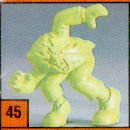x x x x x x x x 46. Invisible Man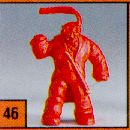x x x x x x x x 47. Skeletonx x x x x x x x 48. Hunchback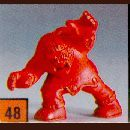x x x x x x x x